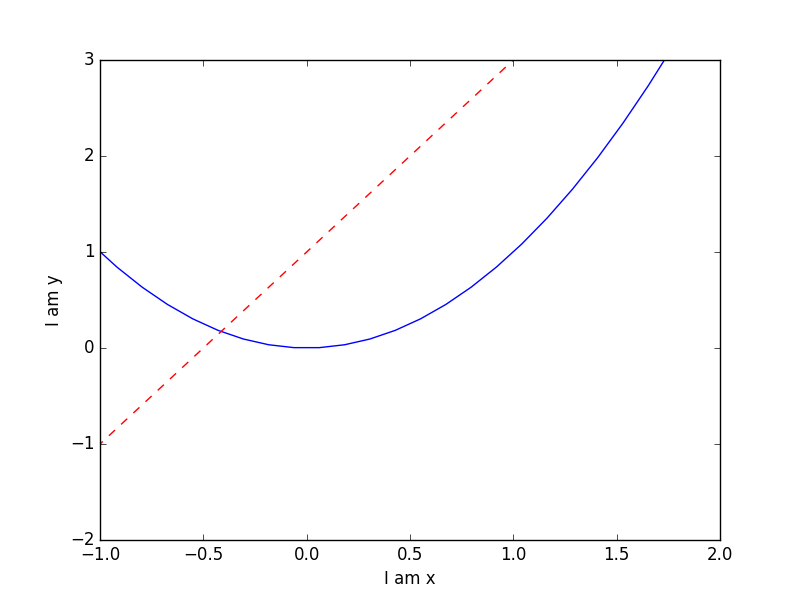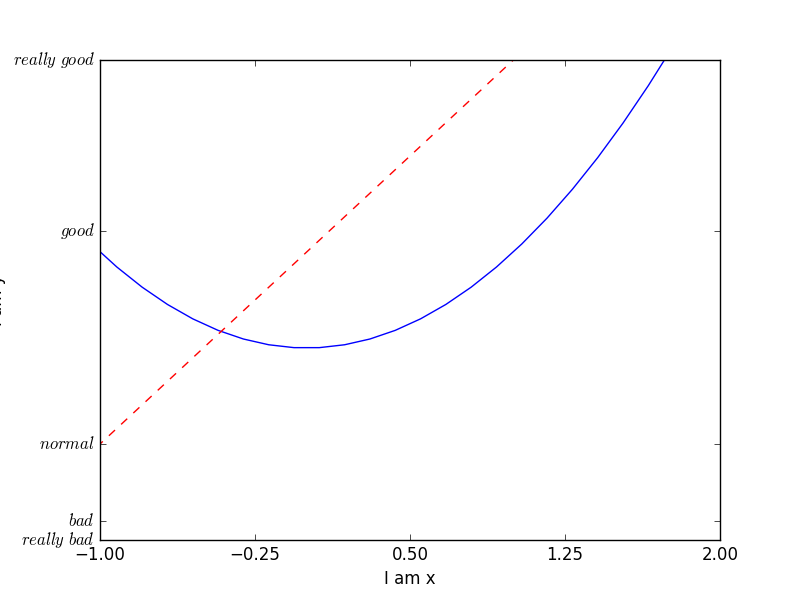# matplotlib 设置坐标轴1

439℃

## 调整名字和间隔

``````import matplotlib.pyplot as plt
import numpy as np``````

``````x = np.linspace(-3, 3, 50)
y1 = 2*x + 1
y2 = x**2``````

``````plt.figure()
plt.plot(x, y2)
plt.plot(x, y1, color='red', linewidth=1.0, linestyle='--')``````

``````plt.xlim((-1, 2))
plt.ylim((-2, 3))
plt.xlabel('I am x')
plt.ylabel('I am y')
plt.show()````````````new_ticks = np.linspace(-1, 2, 5)
print(new_ticks)
plt.xticks(new_ticks)``````

``````plt.yticks([-2, -1.8, -1, 1.22, 3],[r'\$really\ bad\$', r'\$bad\$', r'\$normal\$', r'\$good\$', r'\$really\ good\$'])
plt.show()``````